Hostname: page-component-5db6c4db9b-v64r6 Total loading time: 0 Render date: 2023-03-25T15:25:18.850Z Has data issue: true Feature Flags: { "useRatesEcommerce": false } hasContentIssue true

## Abstract

In this paper, we consider the problem $-\Delta u =-u^{-\beta }\chi _{\{u>0\}} + f(u)$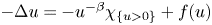in $\Omega$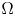with $u=0$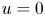on $\partial \Omega$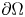, where $0<\beta <1$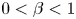and $\Omega$is a smooth bounded domain in $\mathbb {R}^{N}$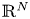, $N\geq 2$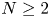. We are able to solve this problem provided $f$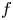has subcritical growth and satisfy certain hypothesis. We also consider this problem with $f(s)=\lambda s+s^{\frac {N+2}{N-2}}$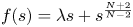and $N\geq 3$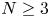. In this case, we are able to obtain a solution for large values of $\lambda$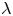. We replace the singular function $u^{-\beta }$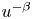by a function $g_\epsilon (u)$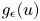which pointwisely converges to $u^{-\beta }$as $\epsilon \rightarrow 0$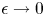. The corresponding energy functional to the perturbed equation $-\Delta u + g_\epsilon (u) = f(u)$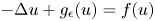has a critical point $u_\epsilon$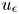in $H_0^{1}(\Omega )$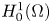, which converges to a non-trivial non-negative solution of the original problem as $\epsilon \rightarrow 0$.

Type
Research Article
Information

## Access options

Get access to the full version of this content by using one of the access options below. (Log in options will check for institutional or personal access. Content may require purchase if you do not have access.)

## References

Ambrosetti, A., Brezis, H. and Cerami, G., Combined effects of concave and convex nonlinearities in some elliptic problems, J. Funct. Anal. 122 (1994), 519543.CrossRefGoogle Scholar
Anello, G. and Faraci, F., On a resonant elliptic problem with singular terms, Nonlinear Anal. 195 (2020), 111818.CrossRefGoogle Scholar
Aris, R., The mathematical theory of diffusion and reaction in permeable catalysts (Clarendon Press, 1975).Google Scholar
Bai, Y., Gasiński, L. and Papageorgiou, N. S., Positive solutions for nonlinear singular superlinear elliptic equations, Positivity 23 (2019), 761778.CrossRefGoogle Scholar
Brezis, H. and Nirenberg, L., Positive solutions of nonlinear elliptic equations involving critical Sobolev exponents, Comm. Pure Appl. Math. 36 (1983), 437477.CrossRefGoogle Scholar
Copetti, M. I. M. and Elliott, C. M., Numerical analysis of the Cahn-Hilliard equation with a logarithmic free energy, Numer. Math. 63 (1992), 3965.CrossRefGoogle Scholar
Costa, D. G., An invitation to variational methods in differential equations (Birkhäuser, 2007).CrossRefGoogle Scholar
Dal Passo, R., Giacomelli, L. and Novick-Cohen, A., Existence for an Allen-Cahn/Cahn-Hilliard system with degenerate mobility, Interfaces Free Bound 1 (1999), 199226.CrossRefGoogle Scholar
Dávila, J. and Montenegro, M., Positive versus free boundary solutions to a singular elliptic equation, J. Anal. Math. 90 (2003), 303335.CrossRefGoogle Scholar
Dávila, J. and Montenegro, M., Concentration for an elliptic equation with singular nonlinearity, J. Math. Pures Appl. 97 (2012), 545578.CrossRefGoogle Scholar
Diaz, J. I., Nonlinear partial differential equations and free boundaries, Volume I. Elliptic equations, Research Notes in Mathematics, Vol. 106 (Boston, Pitman, 1985).Google Scholar
Diaz, J. I., Morel, J. M. and Oswald, L., An elliptic equation with singular nonlinearity, Commun. Partial Differ. Equ. 12 (1987), 13331344.CrossRefGoogle Scholar
Elliot, C. M. and Garcke, H., On the Cahn-Hilliard equation with degenerate mobility, SIAM J. Math. Anal. 27 (1996), 404423.CrossRefGoogle Scholar
Figueiredo, G. and Montenegro, M., A class of elliptic equations with singular and critical nonlinearities, Acta Appl. Math. 143 (2016), 6389.CrossRefGoogle Scholar
Friedman, A. and Phillips, D., The free boundary of a semilinear elliptic equation, Trans. AMS 282 (1984), 153182.CrossRefGoogle Scholar
Gilardi, G. and Rocca, E., Well-posedness and long-time behaviour for a singular phase field system of conserved type, IMA J. Appl. Math. 72 (2007), 498530.CrossRefGoogle Scholar
Hirano, N., Saccon, C. and Shioji, N., Existence of multiple positive solutions for singular elliptic problems with concave and convex nonlinearities, Adv. Differ. Equ. 9 (2004), 197220.Google Scholar
Lions, P. L., The concentration-compactness principle in the calculus of variations. The limit case, Rev. Mat. Iberoamericana 1 (1985), 145201.CrossRefGoogle Scholar
Long, Y. M., Sun, Y. J. and Wu, S. P., Combined effects to singular and superlinear nonlinearities in some singular boundary value problems, J. Differ. Equ. 176 (2003), 511531.Google Scholar
Lorca, S. and Montenegro, M., Free boundary solutions to a log-singular elliptic equation, Asymptot. Anal. 82 (2013), 91107.Google Scholar
Montenegro, M. and Queiroz, O., Existence and regularity to an elliptic equation with logarithmic nonlinearity, J. Differ. Equ. 246 (2009), 482511.CrossRefGoogle Scholar
Montenegro, M. and Silva, E. A. B., Two solutions for a singular elliptic equation by variational methods, Ann. Scuola Normale Sup. Pisa 11 (2012), 143165.Google Scholar
Papageorgiou, N. S. and Rădulescu, V., Combined effects of singular and sublinear nonlinearities in some elliptic problems, Nonlinear Anal. 109 (2014), 236244.CrossRefGoogle Scholar
Papageorgiou, N. S. and Smyrlis, G., A bifurcation-type theorem for singular nonlinear elliptic equations, Methods Appl. Anal. 22 (2015), 147170.CrossRefGoogle Scholar
Perera, K. and Silva, E. A. B., Existence and multiplicity of positive solutions for singular quasilinear problems, J. Math. Anal. Appl. 323 (2006), 12381252.CrossRefGoogle Scholar
Phillips, D., A minimization problem and the regularity of solutions in the presence of a free boundary, Indiana Univ. Math. J. 32 (1983), 117.CrossRefGoogle Scholar
Shi, J. and Yao, M., On a singular nonlinear semilinear elliptic problem, Proc. Roy. Soc. Edinburgh Sect. A 128 (1998), 13891401.CrossRefGoogle Scholar
Wang, X., Qin, X. and Hu, G., Existence of weak positive solution for a singular elliptic problem with supercritical nonlinearity, Anal. Math. Phys. 8 (2018), 4355.CrossRefGoogle Scholar
Willem, M., Minimax theorems (Birkhäuser, 1996).CrossRefGoogle Scholar
Zhang, Z., On a Dirichlet problem with a singular nonlinearity, J. Math. Anal. Appl. 194 (1995), 103113.CrossRefGoogle Scholar